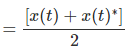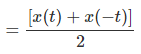Courses

Static & Dynamic Systems Notes | Study Digital Signal Processing - Electrical Engineering (EE)

Electrical Engineering (EE): Static & Dynamic Systems Notes | Study Digital Signal Processing - Electrical Engineering (EE)

The document Static & Dynamic Systems Notes | Study Digital Signal Processing - Electrical Engineering (EE) is a part of the Electrical Engineering (EE) Course Digital Signal Processing.
All you need of Electrical Engineering (EE) at this link: Electrical Engineering (EE)

Some systems have feedback and some do not. Those, which do not have feedback systems, their output depends only upon the present values of the input. Past value of the data is not present at that time. These types of systems are known as static systems. It does not depend upon future values too.

Since these systems do not have any past record, so they do not have any memory also. Therefore, we say all static systems are memory-less systems. Let us take an example to understand this concept much better.

Example

Let us verify whether the following systems are static systems or not.

• y(t) = x(t) + x(t − 1)
• y(t) = x(2t)
• y(t) = x = sin[x(t)]

a) y(t) = x(t) + x(t − 1)

Here, x(t) is the present value. It has no relation with the past values of the time. So, it is a static system. However, in case of x(t-1), if we put t = 0, it will reduce to x(-1) which is a past value dependent. So, it is not static. Therefore here y(t) is not a static system.

b) y(t) = x(2t)

If we substitute t = 2, the result will be y(t) = x(4). Again, it is future value dependent. So, it is also not a static system.

c) y(t) = x = sin[x(t)]

In this expression, we are dealing with sine function. The range of sine function lies within -1 to +1. So, whatever the values we substitute for x(t), we will get in between -1 to +1. Therefore, we can say it is not dependent upon any past or future values. Hence, it is a static system.

From the above examples, we can draw the following conclusions −

• Any system having time shifting is not static.
• Any system having amplitude shifting is also not static.
• Integration and differentiation cases are also not static.

If a system depends upon the past and future value of the signal at any instant of the time then it is known as dynamic system. Unlike static systems, these are not memory less systems. They store past and future values. Therefore, they require some memory. Let us understand this theory better through some examples.

Examples

Find out whether the following systems are dynamic.

a) y(t) = x(t+1)

In this case if we put t = 1 in the equation, it will be converted to x(2), which is a future dependent value. Because here we are giving input as 1 but it is showing value for x(2). As it is a future dependent signal, so clearly it is a dynamic system.

b) y(t) = Real[x(t)]In this case, whatever the value we will put it will show that time real value signal. It has no dependency on future or past values. Therefore, it is not a dynamic system rather it is a static system.

c) y(t) = Even[x(t)]Here, if we will substitute t = 1, one signal shows x(1) and another will show x(-1) which is a past value. Similarly, if we will put t = -1 then one signal will show x(-1) and another will show x(1) which is a future value. Therefore, clearly it is a case of Dynamic system.

d) y(t) = cos[x(t)]

In this case, as the system is cosine function it has a certain domain of values which lies between -1 to +1. Therefore, whatever values we will put we will get the result within specified limit. Therefore, it is a static system

From the above examples, we can draw the following conclusions −

• All time shifting cases signals are dynamic signals.
• In case of time scaling too, all signals are dynamic signals.
• Integration cases signals are dynamic signals.
The document Static & Dynamic Systems Notes | Study Digital Signal Processing - Electrical Engineering (EE) is a part of the Electrical Engineering (EE) Course Digital Signal Processing.
All you need of Electrical Engineering (EE) at this link: Electrical Engineering (EE)Use Code STAYHOME200 and get INR 200 additional OFF Use Coupon Code

Top Courses for Electrical Engineering (EE)Digital Signal Processing

3 videos|50 docs|54 tests

Top Courses for Electrical Engineering (EE)Track your progress, build streaks, highlight & save important lessons and more!

,

,

,

,

,

,

,

,

,

,

,

,

,

,

,

,

,

,

,

,

,

;